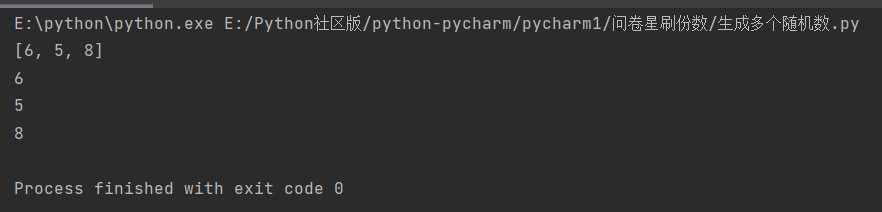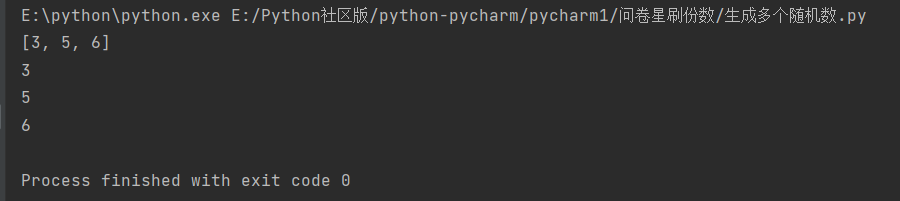• python生成多个随机数
2020-11-11 13:51:48

你可以从上面发布的mtrw中加快一点，只需要做最初描述的内容(生成一堆随机数，并相应地进行相乘和分割)…

此外，您可能已经知道了这一点，但是在使用大型的ish numpy数组时，请务必在现场(* =，/ =，=等)中进行操作。它与大型阵列的内存使用量有很大的差异，同时也会大大提高速度。

In : def rand_row_doubles(row_limits, num):

....: ncols = len(row_limits)

....: x = np.random.random((num, ncols))

....: x *= row_limits

....: return x

....:

In : %timeit rand_row_doubles(np.arange(7) + 1, 1000000)

10 loops, best of 3: 187 ms per loop

相比之下：

In : %timeit ManyRandDoubles(np.arange(7) + 1, 1000000)

1 loops, best of 3: 222 ms per loop

这不是很大的区别，但如果你真的担心速度，那就是事情。

只是为了表明这是正确的：

In : x.max(0)

Out:

array([ 0.99999991, 1.99999971, 2.99999737, 3.99999569, 4.99999836,

5.99999114, 6.99999738])

In : x.min(0)

Out:

array([ 4.02099599e-07, 4.41729377e-07, 4.33480302e-08,

7.43497138e-06, 1.28446819e-05, 4.27614385e-07,

1.34106753e-05])

同样，对于你的“行总和到一个”部分…

In : def rand_rows_sum_to_one(nrows, ncols):

....: x = np.random.random((ncols, nrows))

....: y = x.sum(axis=0)

....: x /= y

....: return x.T

....:

In : %timeit rand_rows_sum_to_one(1000000, 13)

1 loops, best of 3: 455 ms per loop

In : x = rand_rows_sum_to_one(1000000, 13)

In : x.sum(axis=1)

Out: array([ 1., 1., 1., ..., 1., 1., 1.])

老实说，即使你在C中重新实现，我也不确定你能在这一点上能够打败多少，但是我可能是非常错误的！

更多相关内容
• 本文实例讲述了Python简单生成随机数的方法。分享给大家供大家参考，具体如下： 主要知识点： 随机整数： random.randint(a,b)：返回随机整数x,a<=x<=b 包含范围的随机整数 random.randrange(start,stop,[,...
• 目录 一、前言 二、正文 1.函数介绍 2.代码解析 ...在上篇博文中，我们实现了问卷星的单选功能，对于多选的功能，... random.randint(m, n)表示返回一[m,n]的随机整数。 ②函数sort()用于列表中元素的排列，不...

目录

一、前言

二、正文

1.函数介绍

2.代码解析

三、总结

# 一、前言

在上篇博文中，我们实现了问卷星的单选功能，对于多选的功能，需要学习一下这篇博文的内容。

隔壁寝室刷问卷刷疯了，我们寝室则相反，打游戏的同时问卷份数也在增加，隔壁寝室的人投来羡慕的目光，向我要代码！！

# 二、正文

## 1.函数介绍

①random模块中的random.randint()函数

random.randint(m, n)表示返回一个[m,n]的随机整数。

函数sort()用于列表中元素的排列，不写任何参数则会将列表中的元素升序（从小到大）排列。

## 2.代码解析

代码如下

import random
def int_random(m, n, o):
p = []
while len(p) < o:
new_int = random.randint(m, n)
if (new_int not in p):
p.append(new_int)
else:
pass
return p
q = int_random(1, 4, 3)
print(q)
# 遍历列表内容，并打印
for r in q:
print(r)


字母解释：m表示生成的随机数的下限，n表示生成的随机数的上限，o表示生成随机数的个数。

思路：

①首先定义一个函数int_random()，创建一个空列表p，如果列表p里面的个数少于你所写的o（生成随机数的个数），随机生成一个在m和n之间（包括m，n）的的一个数，接下来的if函数可以用来去重复数字。如果新生成的数字列表中已经有了，则跳过这个，重新进入while循环，重新生成随机数，反之，则在列表p中添加这个随机数。最后这个int_random()函数返回的结果是列表p。

②for循环表示遍历列表p的内容。

③m，n，o的内容可以自定，只要满足o在m和n之间就好。

注：while len(p) < o中的<不能写成<=，否则会使得多一个随机数，如果o和n相等时，会长时间运行而不报错。

④以上代码生成的列表和遍历的内容是无序的，要将它升序排列，只需用到sort()函数，

import random
def int_random(m, n, o):
p = []
while len(p) < o:
new_int = random.randint(m, n)
if (new_int not in p):
p.append(new_int)
else:
pass
return p
q = int_random(3, 8, 3)
q.sort()
print(q)
# 遍历列表内容，并打印
for r in q:
print(r)


只需在q = int_random(3, 8, 3)后面加一个 q.sort()就可以实现元素有序排列。

未加sort()函数加了sort()函数# 三、总结

这篇文章主要讲述生成多个随机数的思路。在下篇文章中我们将会将它应用到问卷星问卷的多选题上，如果觉得本文写的不错的，欢迎点赞、评论，您的想法将会是我努力的动力！！！

展开全文• # 生成数字 def rand_num(): return str(random.randint(0, 9)) # 生成大写字母 def rand_upper(): return chr(random.randint(65, 90)) # 生成小写字母 def rand_lower(): return chr(random.randint(97, 122)...
# 生成数字
def rand_num():
return str(random.randint(0, 9))

# 生成大写字母
def rand_upper():
return chr(random.randint(65, 90))

# 生成小写字母
def rand_lower():
return chr(random.randint(97, 122))

# 生成n位随机验证码
def rand_verify_code(n=4):
lst = ["rand_num", "rand_upper", "rand_lower"]
str = ""
for i in range(n):
str += (eval(random.choice(lst))())
return str

n = int(input('输入验证码的位数: '))
print(rand_verify_code(n))

输入验证码的位数: 9
vN87ZgpQY

展开全文pycharm 算法
• python如何一次性取出多个随机数python 如何生成和为固定值的N个随机数？例如 我需要生成一组随机数，要CSS布局HTML小编今天和大家分享所有随机数的和为 60，随机数的个数为1很简单，不用那么蠢的代码。 如果你不...

python如何一次性取出多个随机数

python 如何生成和为固定值的N个随机数？

例如 我需要生成一组随机数，要CSS布局HTML小编今天和大家分享所有随机数的和为 60，随机数的个数为1很简单，不用那么蠢的代码。 如果你不需要最终产生的随机数是整数的话，只需要随机产生10个随机数，然后计算它们的合是多少，然后算下这个合和60之间的比例，把所有的随机数乘以一个比例就可以了。给你两个方法参考，都是可以的。python里面如何生成随机数？

今天的python培训作业，CSS布局HTML小编今天和大家分享帮助，最好详细一点～

random模块 随机整数：random.randint(a,b)：返回随机整数x,a

怎么样用python做个程序。生成一个随机数构成的列表

请高手指教下。怎么样用python做个程序。生成一个随机数构成的列表？？ 概述利用python的random包和list列表 代码详解首先导入random包 这里选择获取键入的数值来作为随机列表的长度和随机列表的取值范围 由于python支持表达式作参数这里简写了一点 import random length = int(input("请输入你需要获得的列表长度"))

用python生成随机数的几种方法

1 从给定参数的正态分布中生成随机数 当考虑从正态分布中生成随机数时，应当首先知道正态分布的均值和方差(标准差)&

展开全文• 您可以使用Format-Preserving ... 对于Python中的另一例子，使用另一非AES FFX（我认为）方法，请参见this blog post "How to Generate an Account Number"，它使用Feistel密码执行FPE。它生成从0到2^32-1的数字。
• 原博文2019-01-12 12:51 −有些时候需要发送短信给用户生成四位随机数字，这里在python中我们可以根据python自带的标准库random和string来实现。 random下有三可以随机取数的函数，分别是choice，choices，sample ...
• 如果你对在Python生成随机数与random模块中最常用的几函数的关系与不懂之处，下面的文章就是对Python生成随机数与random模块中最常用的几函数的关系，希望你会有所收获，以下就是这篇文章的介绍。random.random...
• python中的random模块用于生成随机数，random模块中有很方法，其中randint想要使用 Python 生成随机不重复的数，我们可以使用 random 模块来实现：>>> import random## 先创 list>>> list = [1.0 ,1.2 ,1.4, 1.3...
• 随机数在我们的生产和生活中有很的应用场景，比如说登录验证的随机数字等等，那么你知道在Python中怎么生成随机数吗？往下看，就是这么简单！题目python生成随机整数、随机小数、0--1之间小数方法代码先上代码~...
• 主要介绍了Python编程实现生成特定范围内不重复多个随机数的2种方法,涉及Python基于random生成随机数的常见操作技巧,需要的朋友可以参考下
• python如何一次性取出多个随机数python生成随机数的几种方法1 从给定参数的正态分布中生成随机数 当考虑从正态分布中生成随机数时，应当首先知道正态分布的均值和方差(标准差)，有了这些，就可以调用python中现有...
• >>> import random>>> random.randint(100000000000,999999999999)544234865004L如果你想用前导零，你需要一字符串。>>> "%0.12d" % random.randint(0,999999999999)'023432326286'编辑：我自己解决这问题的方法...
• numpy 中 的random模块有多个函数用于生成不同类型的随机数，常见的有 uniform、rand、random、randint、random_interges 下面介绍一下各自的用法 1、np.random.uniform的用法 np.random.uniform(low=0.0, high...
• 15 print(random.choice(['剪刀', '石头', '布'])) # 随机选取字符串 16 print(random.randrange(1, 100, 2)) # 生成从1到100的间隔为2的随机整数 17 print(random.sample('zyxwedcba', 5)) # 多个字符中生成指定...
• 首先,需要导入random模块:import random随机取1-33之间的1个随机数，可能重复:random.choice(range(1,34))print得到一系列随机数,执行一次得到一个随机数:print(random.choice(range(1,34)))随机取1-33之间的6...
• python生成不同随机数的方法发布时间：2020-09-02 10:20:32来源：亿速云阅读：60作者：小新这篇文章主要介绍用python生成不同随机数的方法，文中介绍的非常详细，具有一定的参考价值，感兴趣的小伙伴们一定要看完...
• 一、生成随机数种子 np.random.seed(0) 二、生成aaa到bbb的长度为nnn的数列 import numpy as np np.random.randint(a,b + 1,n)
• import randomimportstringimport timefromdatetime import date,timedeltaclassrandoms():# 获取26大小写字母letters= string.ascii_letters# 获取26小写字母Lowercase_letters= string.ascii_lowercase# 获取...
• python生成随机数、随机字符串import randomimport string# 随机整数：print random.randint(1,50)# 随机选取0到100间的偶数：print random.randrange(0, 101, 2)# 随机浮点数：print random.random()print random....
• 困难的部分是,在运行时之前,我将不知道需要多少个数字我有一个程序,一次使用一个随机数,但它需要执行次.到目前为止,我尝试过的事情是：>使用random.random()一次生成个随机数>使用np.random.rand()一次生成...
• 第二种：通过 Random 类来产生一个随机数。第三种：通过 System.currentTimeMillis() 来获取一个当前时间毫秒数的 long 型数字。Java指定位数随机数通过以上三种方式，可演变的使用Java生成指定位数随机数的方式就有...
• 你可以把它和for循环一起使用10次random.randint(10, 90)将给你一10到90之间的随机数(不是一列表)有了这些信息，我们可以将脚本更改为：import randomeven_sum = 0odd_sum = 0for number_of_turns in r...
• Python循环语句 编写程序时的三种顺序： 顺序:从上向下,顺序执行代码 分支:根据条件判断,决定执行代码的分支 循环:让特定代码重复执行(解决程序员重复工作) Python for循环语句 for 变量 in range():、 range...
• import numpy as np calc = True while calc: n = np.random.rand(3)*1000 n = n.round(0) calc = not n.sum() == 2000 print(n, n.sum())开发语言...